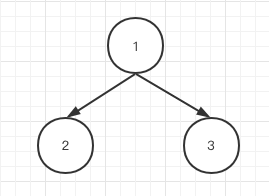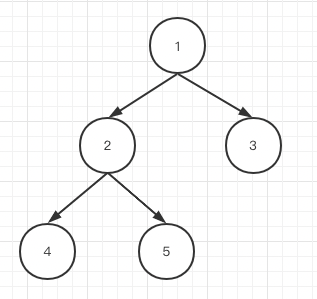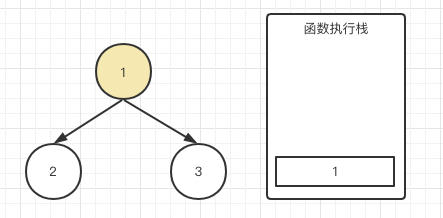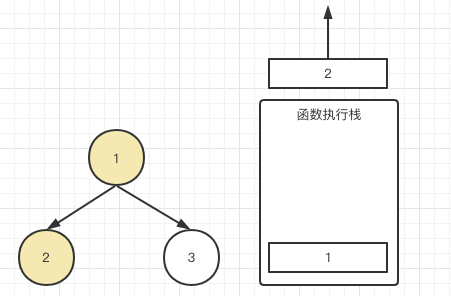# 听风是风

## 五二不休息，今天也学习，从JS执行栈角度图解递归以及二叉树的前、中、后遍历的底层差异# 壹 ❀ 引

const traverse = (root) => {
if (root === null) {
return;
};
// 前序遍历
traverse(root.left);
// 中序遍历
traverse(root.right);
// 后序遍历
}


# 贰 ❀ 从递归说起

• 函数得自己调用自己
• 你只用关注函数第一次调用应该做什么，不需要关注之后递归做什么，因为递归会帮你做第一次相同的操作
• 你需要考虑什么条件下终止递归
• 每次递归是否需要设置返回值？不返回能不能解决当前问题

const arr = [1, 2, 3];
// 正序遍历
let i = 0
// 跳出条件
while (i < arr.length) {
// 当前要做什么？
console.log(arr[i]); // 3 2 1
i++;
};


• 自己调用自己不用说了，硬性条件
• 每一步只是做console.log(arr[i])以及让i自增
• 正序遍历，当i = arr.length时就得跳出递归
• 没有返回值，不需要考虑

const traverse = (arr, i = 0) => {
// 跳出条件
if (i >= arr.length) return;
// 第一次调用做什么？输出arr[i];
console.log(arr[i]); // 1 2 3
// 自己调用自己
traverse(arr, i + 1);
};
traverse(arr);// 倒序遍历
let i = arr.length - 1;
// i>=0我还能继续做一件事
while (i >= 0) {
console.log(arr[i]); // 3 2 1
i--;
};


const traverse = (arr, i = arr.length - 1) => {
// i<0我不能继续做一件事
if (i < 0) return;
// 第一次调用做什么？输出arr[i];
console.log(arr[i]); // 1 2 3 4 5 6
// 自己调用自己
traverse(arr, i - 1);
};
traverse(arr);


# 叁 ❀ 执行栈的先进后出

const traverse = (arr, i = 0) => {
if (i >= arr.length) return;
traverse(arr, i + 1);
console.log(arr[i]); // 3 2 1
};
traverse(arr);


1. i=0时，满足条件，结果遇到了traverse(arr, i + 1)，这时候创建一个新的函数上下文，且这个函数不执行完毕，后面的console.log根本无法执行，所以等着。
2. i=1时，同上，又遇到了traverse(arr, i + 1)，继续创建新的函数上下文，第二个函数的console.log也等着了。
3. i=2时，同上，又遇到traverse(arr, i + 1)，继续创建新的上下文。
4. i=3时，不满足条件直接返回，执行完毕，此时是不是表示i=2traverse执行完成了，那么i=2console.log不就可以执行了，于是第一输出了3
5. i=2创建的上下文执行完毕，标注i=1traverse执行完了，它也能执行后面的console.log
6. 于是输出了3 2 1

• 不会写递归？那就写好while，将while的条件改成相反就是递归的跳出条件，因为while是满足什么条件做一件事，递归是不满足某个条件不做一件事，之后将while做的事照搬给递归即可，这里不需要考虑这件事应该放在递归前还是后。
• i同等自增或自减情况下，可以将做一件事的行为放在递归调用的前或者后，便能达到正序或倒序遍历的效果。
• 正序和倒序的底层差异其实就是执行栈的先进后出，正序是先进（先把事做了再进），倒序是后出（啥也别做先进，出的时候再做一件事）

# 肆 ❀ 用执行栈看二叉树前、中、后序遍历差异const traverse = (root) => {
if (root === null) {
return;
};
console.log(root.val);
traverse(root.left);
traverse(root.right);
}const traverse = (root) => {
if (root === null) {
return;
};
traverse(root.left);
traverse(root.right);
console.log(root.val);
}# 伍 ❀ 总

posted on 2022-05-02 21:43  听风是风  阅读(375)  评论(0编辑  收藏  举报